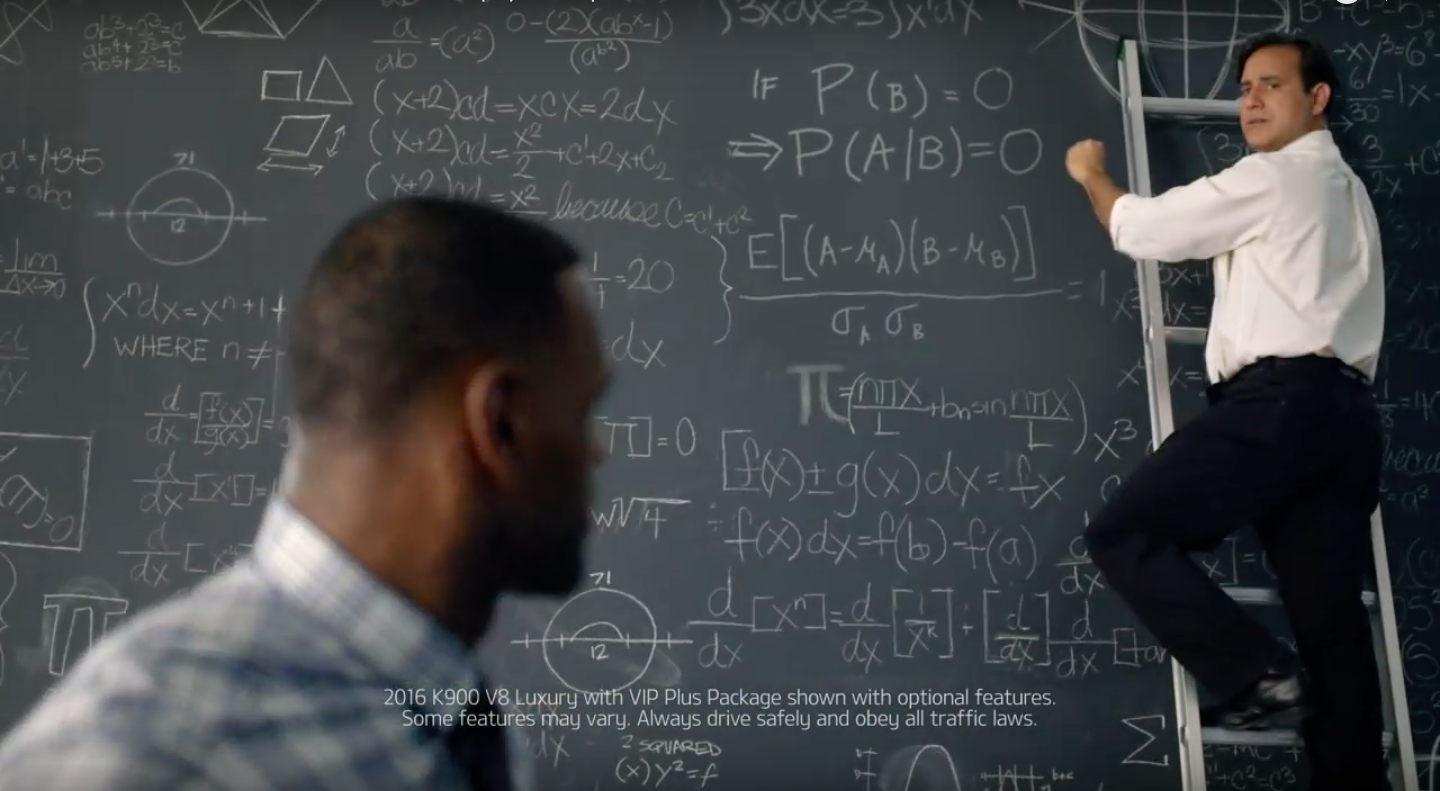# LeBron James and conditional probability

If you’ve been watching the NBA playoffs, you’ve seen this commercial. It, and another Twitter-centric one, show up several times in every game.

Maybe sponsors have decided that having more than two or three commercials in rotation at any given time dilutes their branding. Or maybe ad budgets are tighter than they used to be. But let’s not talk about trends in marketing, let’s talk about conditional probability.

As he walks out of his curiously appointed office, LeBron says

The probability of Event B occurring, given that Event A never occurs is obviously zero.

and the camera shifts focus to the nerd at the blackboard.Let’s ignore LeBron’s mistake—he was denied the opportunity to take a probability course in college. But what the hell is the nerd doing? In conventional notation, what LeBron said was

$P\:(B \;|\, \bar A) = 0$

but what the nerd wrote is

$P\:(A \;|\, B) = 0$

If the makers of the commercial have enough familiarity with conditional probability to get the vertical bar notation correct, how did they put the condition on the wrong side of the bar? And how did they miss using an overbar to represent “not A”? Did they try out a version of the commercial with the right equation and find that it was less funny? That’s hard to believe.

By the way, even if you ignore what LeBron says, the contention on the blackboard,

$\textrm{If } P\:(B) = 0, \textrm{then } P\:(A \;|\, B) = 0$

is wrong. Recall that

$P\:(A \;|\, B) = \frac{P\:(A \cap B)}{P\:(B)}$

So if $$P\:(B) = 0$$, then $$P\:(A \;|\, B)$$ has a division by zero, which makes it undefined, not zero.

Michael Jordan would’ve caught that error.